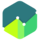COURSE

## Cost of Capital

The cost of capital is the rate of return that a firm pays to bondholders and equity holders. Cost of capital is an important measure while making investment decisions, as any one making an investment would expect a higher return from his investment in a company compared to what he could earn from an alternative investment with equivalent risk.

In this reading, we will learn about how to calculate the weighted average cost of capital, and how marginal cost of capital is used in determining the NPV of a project. We will also learn about how to calculate the cost of each type of capital raised by a company such as debt, preferred stock, and common equity. Finally, we will look at marginal cost of capital schedule, and correct treatment of flotation costs.

#### Course Resources

Study Notes for Cost of Capital
All Users

#### Course Quizzes